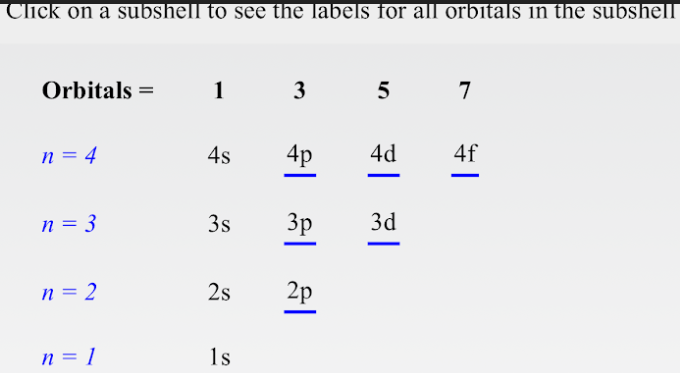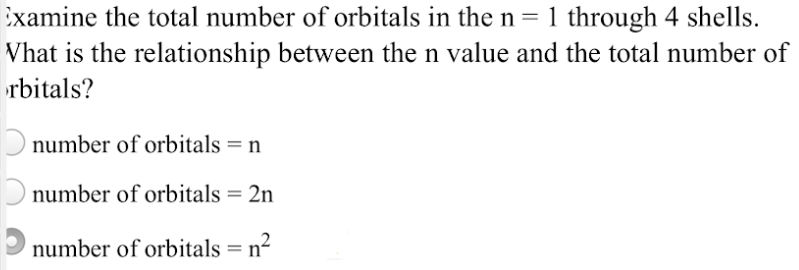# Click on a subshell to see the labels for all orbitals in the subshell. Examine the total number of orbitals in the n = 1 through 4 shells. What is the relationship between the n value and the total number of orbitals? a) number of orbitals = n b) number of orbitals = 2n c) number of orbitals = n^2